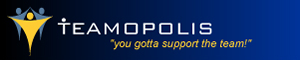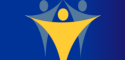# Play Sports Games Online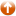Games »  Math »

## O W & L

Most Popular Games: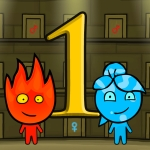Fireboy and Watergirl 1 Forest Temple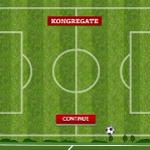SoccerballsWonderputtRugby.io Ball MayhemTiger Simulator 3D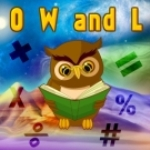4.9
Rate this game:

## O W & L

Plays: 4433

O W and L is a funny math game. This is a puzzle based math game which includes several practices of mathematics with a funny presentation. The base concept is the game is to relate the digits with the word. In this game the word OWL is related to a 3 digit number. In which first letter O represents the first digit of a number. Second letter W represents the second digit of the number and the third letter L represents the third digit of the number. If the number is 245 means that the O is 2, The W is 4 and the L is 5. Now if a question may ask O - W + L = 3, you may choose 245 from the options because it is valid equation with the assumptions of this game. In each question you have to find a number which resembles OWL. For knowing the correct number you will be given some hints. Solve the 50 funny questions of addition, subtraction, multiplication, comparison and algebra to win this game.